# Bihar Board Class 9 Science Solutions Chapter 8 Motion

## BSEB Bihar Board Class 9 Science Solutions Chapter 8 Motion

Bihar Board Class 9 Science Solutions Chapter 8 Motion Textbook Questions and Answers, Additional Important Questions, Notes.

### Bihar Board Class 9 Science Chapter 8 Motion InText Questions and Answers

Page 100

Question 1.
An object has moved through a distance. Can it have zero displacement? If yes, support your answer with an example.Let a car move from A to B and back to A.
∴ Distance = 25 km + 25 km = 50 km Displacement = 0

Question 2.
A farmer moves along the boundary of a tsquare field of side 10 m in 40 s. What will be the magnitude of displacement of the farmer at the end of 2 minutes 20 seconds?
Side of a square = 10 m
Perimeter of square = 4 x 10 m = 40 m
In 40 s the farmer covers 40 m
In 1 s the farmer will cover = $$\frac {40}{40}$$ = 1m
In 80 s (2 min 20 s) the farmer will cover = 1 x 80 = 80 m
Thus, the farmer will come back to the starting point ‘n 80 s after taking two rounds. Hence, displacement is « to.

Question 3.
Which of the following is true for displacement?
(a) It cannot be zero.
(b) Its magnitude is greater than the distance travelled by the object.
(b).

Page 102

Question 1.
Distinguish between speed and velocity.
Speed and velocity differ from each other as follows :
Speed
1. The distance travelled by a moving body per unit time is called the speed.
2. Speed is a scalar quantity.

Velocity:
The distance travelled by a moving body in a particular direction per unit time is called the velocity
Velocity is a vector quantity.Question 2.
Under what conditions is the magnitude of average velocity of an object equal to average speed ?
The magnitude of average velocity is equal to the average speed when the object moves in the same direction along a straight line.

Question 3.
What does the odometer of an automobile measure?
The odometer of an automobile measures the distance covered by the automobile.

Question 4.
What does the path of an object look like when it is in uniform motion?
When an object is in uniform motion it moves along a straight line.

Question 5.
During an experiment, a signal from a spaceship reached the ground station in five minutes. What was the distance of the spaceship from the ground station ? The signal travels at a speed of light that is 3 x 108 ms-1.
Time 5 min – 300 s
Speed = 3 x 108 ms-1
Distance – Speed x Time = 3 x 108 x 300 = 9 x 1010 m

Page 103

Question 1.
When will you say a body is in (i) uniform acceleration (ii) non-uniform acceleration ?
(i) When a body travels in a straight line, and its velocity increases by equal amount in equal intervals of time, it is said to have a uniform accelera-tion.
Examples : (a) A body falling freely under gravity has uniform acceleration.
(b) A ball moving down an inclined plane has uniform acceleration.
(ii) Win the velocity of a body increase’s by unequal amounts equal” intervals of time, it is said to have a non-unifobm acceleration. Example: An auto/car driven on a crowded city road with frequent application of brakes has a non-uniform acceleration.Question 2.
A bus decreases its speed from 80 km/h-1 to 60 km/h-1 in 5 s. Find the acceleration of the bus.
Iritial velocity, υ = 80 km/h
$$\frac {80000}{3600}$$ = 22.22 ms-1
Final velocity,
υ = 60 km/h
$$\frac {60000}{3600}$$
Time, t = 5 s
a = $$\frac {υ – u}{t}$$
= $$\frac{(16.66-22.22) \mathrm{ms}^{-1}}{5 \mathrm{~s}}$$
= – 1.11 ms-2

Question 3.
A train starting from a railway station and moving with uniform acceleration attains a speed 40 km/h-1 in 10 minutes. Find its acceleration.
Initial velocity, u = 0
Final velocity, υ = 40 km/h υ = $$\frac {40000}{3600}$$ = 11.11 ms-1
Time, t = 10 min = 600 s
Acceleration, a = $$\frac {υ – u}{t}$$ = a = $$\frac{(11.11-0) \mathrm{ms}^{-1}}{600 \mathrm{~s}}$$ = 0.018 ms-2

Page 107

Question 1.
What is the nature of the distance-time graph for uniform and non-uniform motion of an object ?
For uniform motion, distance-time graph is a straight line and for non-uniform motion, distance-time graph is a parabola.

Question 2.
What can you say about the motion of an object whose distance-time graph is a straight line parallel to the time axis ?
This indicates that the body is at rest.

Question 3.
What can you say about the motion of an object if its speed-time graph is a straight line parallel to the time axis ?
This indicates that the body is moving at a constant speed with zero acceleration.

Question 4.
What is the quantity which is measured by the area occupied below the velocity-time graph ?
Distance is measured by the area occupied below velocity-time graph.

Page 109

Question 1.
A bus starting from rest moves with a uniform acceleration of 0.1 m s-2 for 2 minutes. Find
(a) the speed acquired.
(b) the distance travelled.
(i) υ = 0
a = 0.1 ms-2
t = 2 min = 120 s
By first equation of motion,
υ = u + at
υ = 0 + 0.1 x 120
υ = 12 ms-1

(ii) By second equation of motion,
s = ut + $$\frac {1}{2}$$ at2
s = O x 120 + $$\frac {1}{2}$$ x 0.1 x (120)2
s = 0 + 0.1 x 60 x 120
s = 720 mQuestion 2.
A train is travelling at a speed of 90 km/h-1 . Brakes are applied so as to produce a uniform acceleration of- 0.5 m s-1 . Find how far the train will go before it is brought to rest.
u = 90 km/h
u = $$\frac {90000}{3600}$$
a = – 0.5 ms-2
υ = 0
By third equation of motion,
2as = υ2 – u2
⇒ 2 x (- 0.5) x s = (0)2 – (25)2
⇒ -1s = -625
⇒ s = 625 m

Question 3.
A trolley, while going down an inclined plane has an acceleration of 2 cm s-2. What will be its velocity 3 s after the start ?
υ = 0
a = 2 cm/s-2
t = 3 s
By first equation of motion,
υ = u + at
⇒ υ = 0 + 2 x 3
⇒ υ = 6 cm/s

Question 4.
A racing car has a uniform acceleration of 4 m s-2. What distance will it cover in 10 s after start ?
υ = 0
a = 4 ms-2
t = 10 s
By second equation of motion,
s = ut + $$\frac {1}{2}$$at-2
s = 0 x 10 + $$\frac {1}{2}$$ x 4 x (10)2
s = 200 mQuestion 5.
A stone is thrown in a vertically upward direction with a velocity of 5 m s-1 . If the acceleration of the stone during its motion is 10 m s-2 in the downward direction, what will be the height attained by the stone and how much time will it take to reach there ?
u = 5 ms-1
a = -10 ms-2
υ = 0
By third equation of motion, 2as v2 – u2
⇒ 2 x (-10) x s = (0)-2 – (5)-2
⇒ – 20 s = – 25
⇒ s = $$\frac {25}{20}$$ = 1.25 m
By first equation of motion,
υ = u + at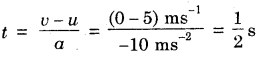t = 0.5 s

### Bihar Board Class 9 Science Chapter 8 Motion Textbook Questions and Answers

Question 1.
An athlete completes one round of a circular track of diameter 200 m in 40 s. What will be the distance and displacement at the end of 2 minutes 20 s?
(i) Distance covered in 40 s
= 2 x π x 100 (r = $$\frac {d}{2}$$ = $$\frac {200m}{2}$$ = 100m)
Distance covered in 1 s = $$\frac {200 π }{40}$$
Distance covered in 2 min 20 s or 140 s
= $$\frac {200 π }{40}$$ x 140
= 5 π x 140 = 700 π = 700 x 3.14 = 2198 m
(ii) Displacement in 2 min 20 s = 0 m (initial and final position is the same).

Question 2.
Joseph jogs from one end A to the other end B of a straight 300 m road in 2 minutes 50 seconds and then turns around and jogs 100 m back to point C in another 1 minute. What are Joseph’s average speeds and velocities in jogging
(a) from A to B and (b) from A to C ?
(a) From A to B
Distance = 300 m
Time = 2 min 50
s = 170 s(b) From A to C
Distance = (300 + 100)m = 400 m
time = (170 + 60 ) s = 230Question 3.
Abdul, while, driving to school computes the average speed for his trip to be 20 km h-1. On his return trip along th same route, there is less traffic and the average speed is 40 km h-1. What is the average speed for Abdul’s trip?
Let the distance between starting point and school be x km.
Average speed from the starting point to school = 20 kmh-1
Time for onward journey = $$\frac {x}{20}$$ h
Average speed from the school to the starting point = 40 kmh-1
Time for return journey = $$\frac {x}{40}$$ h
Average speed for total trip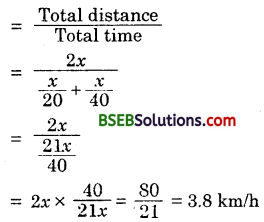Question 4.
A motorboat starting from rest on a lake accelerates in a straight line at a constant rate of 3.0 m s-2 for 8.0 s. How far does the boat travel during this time ?
u = 0
a = 3 ms-2
t = 8 s
According to second equation of motion,
s = ut+ $$\frac {x}{40}$$ at2
s = 0 x 8 + $$\frac {1}{2}$$ x 3 x (8)2
s = 96 mQuestion 5.
A driver of a car travelling at 52 km h-1 applies the brakes and accelerates uniformly in the opposite direction. The car stops in 5 s. Another driver going at 3 km h-1 in another car applies his brakes slowly and stops in 10 s. On the same graph paper, plot the speed versus time graph for two cars. Which of the two cars travelled farther after the brakes were applied ?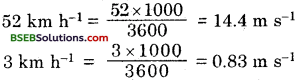At 52 km h-1 the car stops in 5 s At 3 km h-1 the car stops in 10 s.
Distance travelled by first car
= Area of triangle OAB
= $$\frac {1}{2}$$ x OB x OA
= $$\frac {1}{2}$$ x 5 x 14.4 = 36 m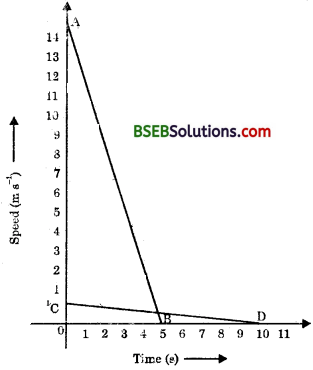Distance travelled by second car = Area of triangle OCD
= $$\frac {1}{2}$$ x OD x OC
= $$\frac {1}{2}$$ x 10 x 0.8 = 4 m.
Thus, the first car travelled farther after the brakes ware applied.

Question 6.
The following figure shows the distance time graph of three objects A, B and C. Study the graph and answer the following questions :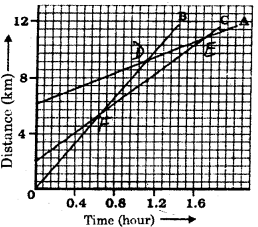(a) Which of the three is travelling the fastest ?
(b) Are all three ever at the same point on the road ?
(c) How far has C travelled when B passes A ?
(d) How far has B travelled by the time it passes C ?
(a) The slope of a distance-time graph gives its speed. The object having the maximum slope will travel the fastest. If we find the slope of A, B and C we will find that B has the greatest slope. Hence, B is travelling fastest.
(b) If the thyee persons are at the same place at a particular time, the three straight lines should intersect at the same point. As there is no such point where the three straight lines intersect, the three persons are never at the same place at the same time.
(c) The graph of A and B intersect at D. (at 1.12 hours). At this time C is at a distance of 8 km.
(d) The graph of B and C intersect and F. This is at a distance,of 5 km.Question 7.
A ball is gently dropped from a height of 20 m. If its velocity increases uniformly at the rate of 10 m s-2 with what velocity will it strike the ground ? After what time will it strike the ground ?
s = 20 m
u = 0
a = 10 m s-2 (since velocity increases at a uniform rate)
By second equation of motion,
s = ut + $$\frac {1}{2}$$ at2
20 = 0 + $$\frac {1}{2}$$ x 10 x t2
⇒ 20 = 5 t-2
$$\frac {1}{2}$$ = t2
or t = $$\sqrt{4}$$ = 2 s
∴ By first equation of motion,
υ = u + at
⇒ υ = 0 + 10 x 2
⇒ υ = 20 ms-2

Question 8.
The speed-time graph for a car is shown in the following figure :(а) Find how far does the car travel in the first 4 seconds. Shade the area on the graph that represents the distance travelled by the car during the period.
(b) Which part of the graph represents uniform motion of the car ?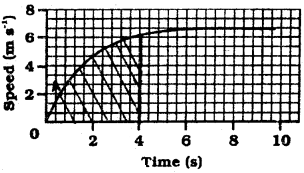(a) In the first 4 seconds,
Speed = 6 m s-1
Distance = Speed x Time
= 6 m s-1 x 4 s = 24m
The shaded portion of the graph represents the distance travelled by the car during the first 4 seconds.
(b) The straight part (OA) of the graph represents uniform motion of the car.

Question 9.
State which of the following situations are possible and give an example of each of these:
(a) a body with a constant acceleration but with zero velocity.
(b) a body moving in a certain direction with an acceleration in vertical direction.
(a) This situation is possible when the body is at rest. (The constant acceleration is zero).
(b) This situation is possible. Example-Motion of a ball thrown horizontally from a height.Question 10.
An artificial satellite is moving in a circular orbit of radius 42250 km. Calculate its – speed if it takes 24 hours to revolve around the earth.
Time (t) = 24 hours 2 nr
υ = $$\frac {2πr}{t}$$
υ = 2 x $$\frac {22}{7}$$ x $$\frac {42250}{24}$$
υ = 11065.47 km/h

### Bihar Board Class 9 Science Chapter 8 Motion Textbook Activities

Activity 8.1

Question 1.
Discuss whether the walls of your classroom are at rest or in motion.
The walls of our classroom are at rest with respect to the immediate environment like a tree or an electric pole. But the walls are in motion with respect to the sun, since the earth moves around the sun.

Activity 8.2

Question 1.
Have you ever experienced that the train in which you are sitting appears to move while it is actually at rest? Discuss.
This generally happens when another train in the next track close to our train is in motion. Hence, our train appears to be moving with respect to the moving train.

Activity 8.3

Question 1.
Take a metre scale and a long rope. Walk from one corner of a basket-ball court to its opposite corner along its sides. Measure the distance covered by you and the magnitude of the displacement. What difference would you notice between the two in this case?
In this case both the distance and the magnitude of displacement will be the same.Activity 8.4

Question 1.
Automobiles are fitted with a device that shows the distance travelled. Such a device is known as an odometer. A car is driven from Bhubaneshwar to New Delhi. The difference between the final reading and the initial reading of the odometer is 1850 km.
Find the magnitude of the displacement between Bhuba-neshwar and New Delhi by using the Road map of India.
Magnitude of displacement can be found as follows :
Draw a right triangle taking Bhubaneswar and New Delhi as two vertices. Measure the hypotenuse of.this triangle. Multiply this value by the scale given in the toad map.

Activity 8.5

The data regarding the motion of two different objects A and B are given in the following table.
Examine them carefully and state whether the motion of the objects is uniform or non-uniform.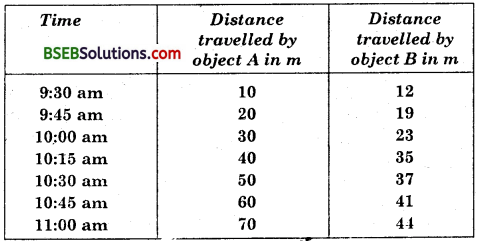The object A covers a distance of 10 m in every fifteen minutes. Thus, it covers equal distance in equal interval of time. Hence the motion of object A is uniform. The object B covers 7 m from 9:30 am to 9:45 am, 4 m from 9:45 am to 10:00 am, 12 m from 10:00 am to 10:15 am. Thus, it covers unequal distance in equal interval of time. Hence the motion of object B is non-uniform.

Activity 8.6

Question 1.
Measure the time it takes you to walk from your house to your bus stop or the school. If you consider that your average walking speed is 4 km h-1estimate the distance of the bus stop or school from your house.
Let the time taken to reach school = 20 min = 1200 s
Speed of walking – 4 km/hr = $$\frac {4000}{3600}$$
Distance = Speed x Time
Distance = ($$\frac {4000}{3600}$$ x 1200) m = 1333 m= 1.33 kmActivity 8.7

At a time when it is cloudy, there may be frequent thunder and lightning. The sound of thunder takes some time to reach you after you see the lightning. Can you answer why this happens? Measure this time interval using a digital wrist watch or a stop watch. Calculate the. distance of the nearest point of lightning. (Speed of sound in air = 346 ms-1)
(i) This is because the speed of light is greater than the speed of sound.
(ii) This time = 30 s
Speed of sound = 346 ms-1
Distance = Speed x Time = 346 x 30 = 10380 m

Activity 8.8

• In your every-day life you come across a range of motions in which

(a) acceleration is in the direction of motion,
(b) acceleration is against the direction of motion,
(c) acceleration is uniform,
(d) acceleration is non-uniform. Can you identify one example of each of the above type of motion?
(a) The motion of a car when it starts from rest and its velocity increases at a steady rate in a particular interval of time.
(b) The motion of a train when it slows down on approaching a station.
(c) The motion of a ball rolling down an inclined plane.
(d) The motion of a car on a busy road.

Activity 8.9

Question 1.
The times of arrival and departure of a train at three stations A, B and C and the distance of stations B and C from station A are given in table below. Plot and interpret the distance-time graph for the train assuming that its motion between any two stations is uniform.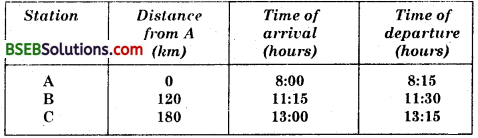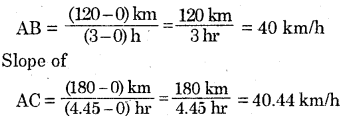.’. The train travels faster from A to C as compared to A to B.

Activity 8.10

Question 1.
Feroz and his sister Sania go to school on their bicycles: Both of them start at the same time from their home but take different times to reach the school although they follow the same route. The following table shows the distance travelled by them in different times. Plot the distance-time graph for their motions on the same scale and interpret.
Table : Distance covered by Feroz and Sania at different times on their bicyclesFrom the graph we see that the speed of Sania is less than the speed of Feroz.Activity 8.11

Question 1.
Take a piece of thread and tie a small piece of stone at one of its ends. Move the stone to describe a circular path with constant speed by holding the thread at the other end.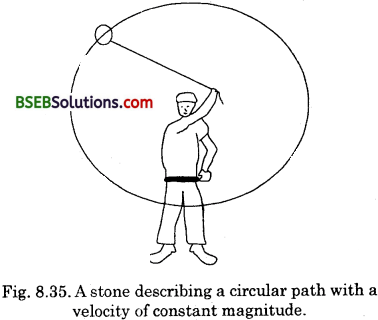Fig. 8.35. A stone describing a circular path with a velocity of constant magnitude.

Now let the stone go by releasing the thread. Can you tell the direction in which the stone moves after it is released ? By repeating the activity for a few times and releasing the stone at different positions of the circular path, check whether the direction in which stone moves remains the same or not ?
The stone will move along a straight line tangentially to the circular path. This is because once the stone is released, it continues to move along the direction it had been moving at that instant. The direction of motion changes at every point while the stone moves along the circular path.

### Bihar Board Class 9 Science Chapter 8 Motion Additional Important Questions and Answers

Question 1.
How do the movements in the living and nonliving objects differ?
The movements in living organisms appear to take place on their own – Nonliving bodies need some outside agency 1,0 set them into motion. For example, to run a car we need the chemical energy of petrol/diesel.

Question 2.
What is motion ? Give some examples of motion in daily life.
(i) A body is said to be in motion if it changes its position in relation to a reference (fixed) point.
(ii) Some examples of motion in daily life are :
(a) Cars moving on the roads.
(b) Aeroplanes flying in the air.
(c) Blades of a fan going round.
(d) Different parts of machines moving in different ways.
(e) Fishes swimming in water.

Question 3.
(i) Give an example of motion in human body which cannot be perceived directly.
(ii) Give an example of motion in nature which cannot be perceived directly.
(iii) Give an example of motion of the earth which cannot be perceived directly.
(i) The motion of blood through veins and arteries in human body cannot be perceived directly.
(ii) We perceive the motion of air by observing the movement of dust, leaves and branches of the trees.
(iii) The phenomena of sunrise, sunset and change of seasons is due to the motion of the earth which cannot be perceived directly.

Question 4.
(i) Give an example of a body which may appear to be moving for one person and stationary for the other.
(ii) What can we tell about motion from the above example ?
(i) The passengers in a moving bus or train observe that the trees, buildings and people on the roadside appear to-be moving backwards. The man standing on the roadside observes that the bus or the train along with its passengers are moving in forward direction. At the same time, each passenger in a moving bus or train observes his fellow sitting passengers are not moving.
(ii) We can tell that motion is relative.Question 1.
What is meant by the term distance?
The actual length of the path covered by the body irrespective of the direction is called the distance. Distance is a scalar quantity.

Question 2.
What is displacement ?.
Change in the position of an object in a particular direction is called displacement. If the position of an object changes from x1 to x2, then the displacement d is given by
d = (x2 – x1)
Displacement is the shortest distance (straight distance) between the initial position and the final position of a moving body.
Displacement is a vector quantity, and it is the distance travelled by a body in a particular direction.

Question 3.
When do we say that the position of a body has changed ?